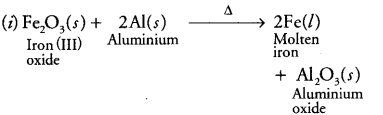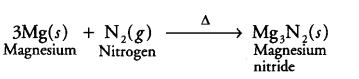# Write the balanced chemical equations for the following reactions and identify the type of reaction in each case. (i) In thermite reaction, iron (III) oxide reacts with aluminium and gives molten iron and aluminium oxide

Write the balanced chemical equations for the following reactions and identify the type of reaction in each case.
(i) In thermite reaction, iron (III) oxide reacts with aluminium and gives molten iron and aluminium oxide.
(ii) Magnesium ribbon is burnt in an atmosphere of nitrogen gas to form solid magnesium nitride.

1 Like

(i)It is a displacement reaction.

(ii)It is a combination reaction.

Bro for 2nd question it is solid magnesium nitrite … What is the equation**
**